# Biasing of Junction Field Effect Transistor or Biasing of JFET

Before going to actual topic let us know what is a pinch-off voltage of a junction field effect transistor because it takes a vital role to decide the biasing level of a junction field effect transistor.

## Pinch Off Voltage

In an n channel JFET, if we apply positive potential at drain terminal keeping the source terminal grounded, there is current from drain to source through the channel due to drift of free electrons from source to drain. This current causes a voltage drop along the channel. By considering this voltage distribution along the channel, we can say that the potential of the channel nearer to the drain terminal is more than that nearer to the source terminal. At the same time if gate terminal is in ground potential, then the PN junction between the gate region and channel becomes reverse biased, and the width of the depletion layer towards drain terminal is more than that of the source terminal.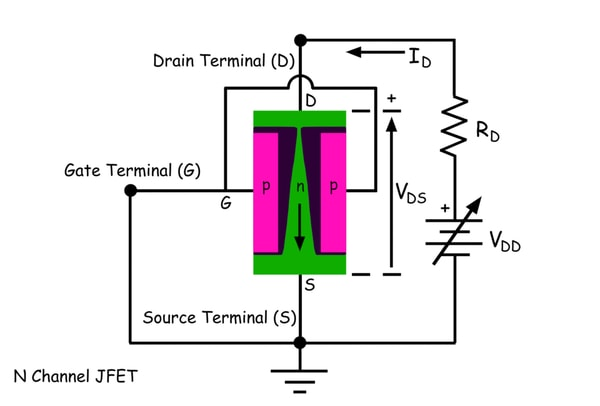Now if we continuously increase the drain voltage, the width of the depletion layer gets increased more rapidly than that towards source terminal. After a certain drain voltage, the depletion layer towards drain terminal touches each other. This voltage is known as pinch-off voltage. That means at zero gate voltage, the drain voltage at which depletion layers from both sides touch together is called pinch-off voltage. It is found that the drain current is linearly proportional to the drain to source voltage before the pinch-off occurs and the drain current becomes almost constant just after pinch-off voltage. If we further increase the drain voltage beyond pinch off voltage the drain current remains constant but after another higher value of drain voltage avalanche breakdown takes place in the reverse biased junction and suddenly drain current rises very rapidly. This voltage is known as the breakdown voltage of JFET. So any junction field effect transistor must be operated between pinch-off voltage and breakdown voltage when it acts as an amplifier. To keep drain to source voltage within the range, a dc voltage source or battery of suitable voltage is connected in series with load resistance or output resistance. The voltage appears between drain and source would be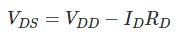The pinch-off voltage appears between drain and source is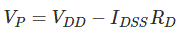Here IDSS is the drain current flowing through the channel at pinch-off while the gate terminal is in ground potential.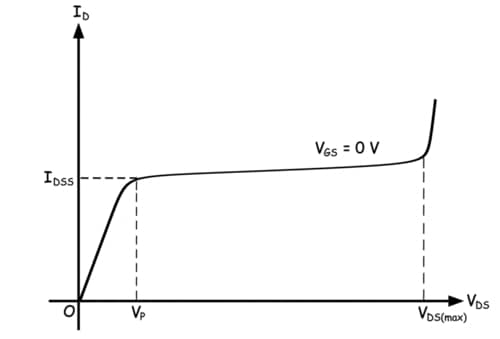Now in n channel JFET, we have to apply negative potential at the gate terminal, and this will further increase the width of the depletion layer between the gate region and channel. Due to the negativity of the p-type region, the reverse biasing of the junction gets increased. It is already discussed that as the drain voltage is so applied keeping gate terminal grounded that the depletion layers towards drain terminal have already been touched and a small channel opening has been created between the layers to allow the drain current to flow.When we increase the negative potential of the gate terminal, the channel opening gets narrower and hence drain current gets reduced. If we go on increasing negative gate terminal voltage, the drain current continues decreasing, and it would be seen that the drain current becomes zero at a certain gate voltage. This voltage is known as gate cut off voltage. The value of the gate cut off voltage is equal to pinch off voltage of a junction field effect, but the polarity of these two voltages are opposite.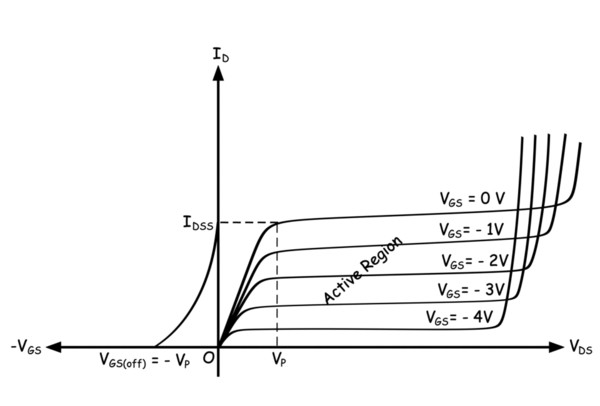So the operating range of the input signal of a JFET should be 0 to – VGS(off) where VGS(off) is the gate cut off voltage. To ensure the operating range of varying input signal the gate circuit must be associated with a fixed biased voltage which can be applied to the gate circuit either by a separate battery source or by voltage diversion from the output circuit. Depending on the applied methods, gate biasing of a JFET can be of three types.

### Biasing of JFET by a Battery at Gate Circuit

This is done by inserting a battery in the gate circuit. The negative terminal of the battery is connected to the gate terminal. As the gate current in JFET is almost zero, there would be no voltage drop across the input gate resistance. Hence the negative potential of the battery directly reaches to gate terminal. The corresponding drain current and drain to source voltage would be the output operating point of the transistor.

NB: – Here in all biasing circuits below, we have included the input AC signal for the better detailing of the circuit, but during calculation of biasing point or operating point of the JFET, we will ignore the AC signal as the biasing only deals with DC.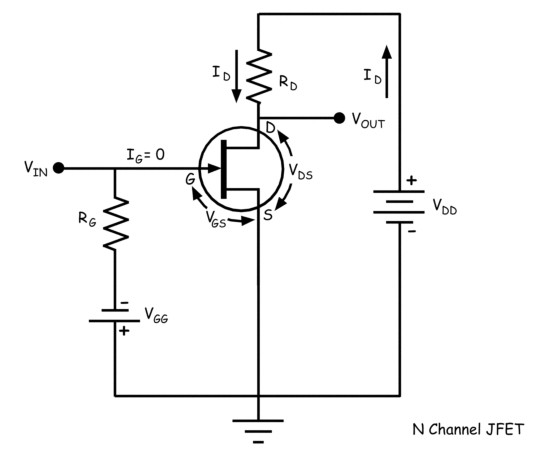As, in JFET there is no gate current,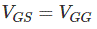We can find the value of drain current ID from the relation given below as IDSS and VGS(off) (= – VP) are given in transistor data sheet.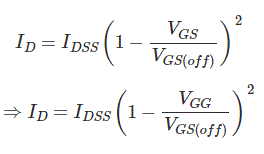The value of VDS can be found by applying KVL at output circuit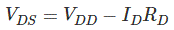The operating point of the JFET is located at the coordinate (VDS, ID) on the characteristic graph.

### Self Biasing of a JFET

Here one resistance RS is inserted between source terminal and ground.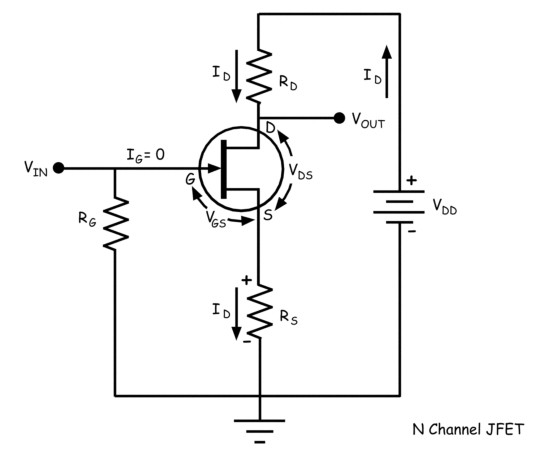The voltage across RS would be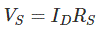Here the gate terminal is also grounded through a resistance RG. As there is no gate current, zero ground potential appears at the gate terminal.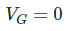The voltage between the gate and source is VGS.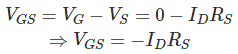This equation tells us that here the gate terminal always gets negative potential than the source terminal.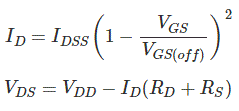After determining the value of ID, and VDS from above relation, we can put the operating point on the characteristic graph at the coordinate (VDS, ID).

### Voltage Divider Biasing of a JFET

Two series connected resistors form a voltage divider circuit. The voltage at the gate terminal can be calculated by voltage division rule. In this way, the applied drain voltage is utilized to get the gate terminal voltage. A resistance is inserted into source terminal in series. The device current flows through the resistance and causes a voltage drop. If this source voltage drop is greater than voltage appears at the gate terminal, the gate to source voltage has a negative value which is desired for JFET operation. Let us consider the following circuit.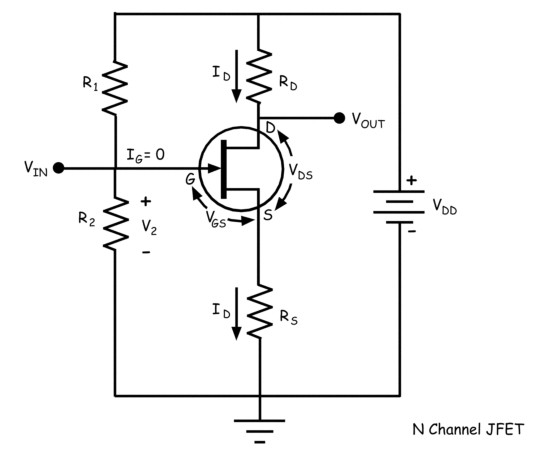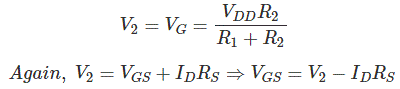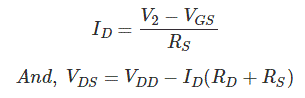Want To Learn Faster? 🎓
Get electrical articles delivered to your inbox every week.
No credit card required—it’s 100% free.